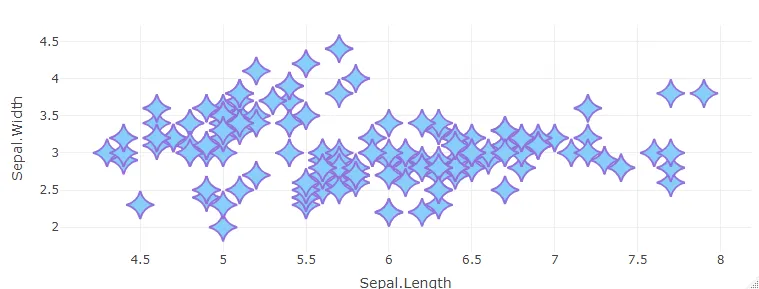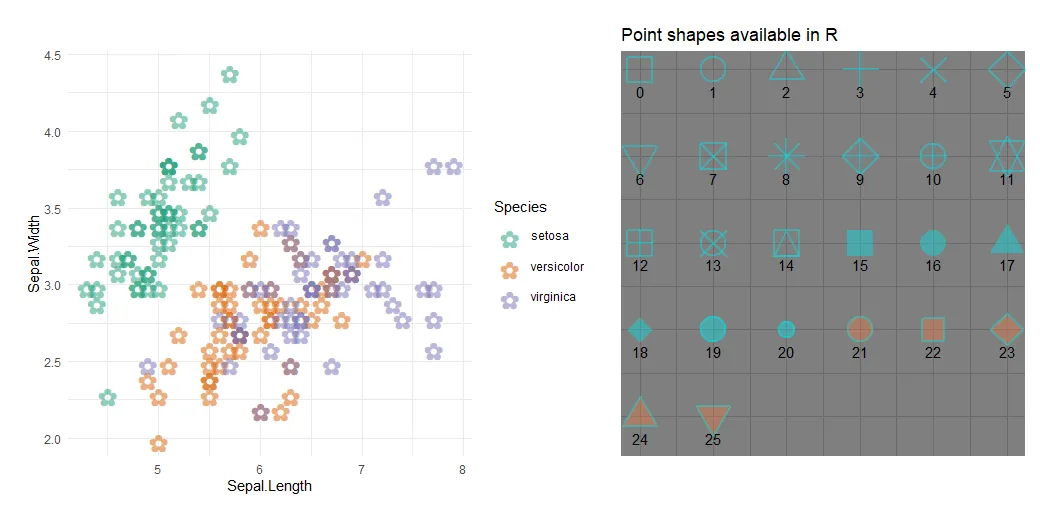# How to change marker shape in the R plot

Sometimes you might wonder how to remove the border around dots in ggplot2, but all you have to do is change the marker shape in the R plot. When creating a plot in R that contains markers, it is possible to adjust the color, transparency, and also shape.

## Look at the marker shapes in R

Here is a way to quickly overlook all the possible point shapes in R.

`ggpubr::show_point_shapes()`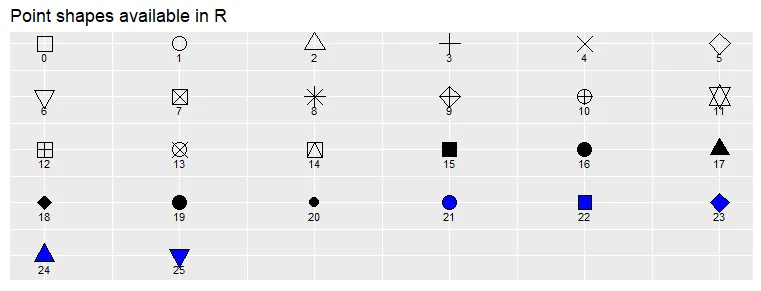If you want to look at different colors for those shapes or compare different formatting options, you can double-click on the function show_point_shapes and modify that code. Here is how it looks.

```require(ggplot2)
require(ggpubr)

d = data.frame(p = c(0:25))

p <- ggplot() +
scale_y_continuous(name = "") +
scale_x_continuous(name = "") +
scale_shape_identity() +
geom_point(data = d,
mapping = aes(x = p%%6, y = p%/%6, shape = p),
size = 6, alpha = 0.4, stroke = 1.5,
color = "turquoise2", fill = "sienna2") +
geom_text(data = d,
mapping = aes(x = p%%6, y = p%/%6 + 0.25, label = p),
size = 4) +
scale_y_reverse() +
theme_dark() +
theme(axis.title = element_blank())

ggpar(p, ticks = FALSE, tickslab = FALSE,
main = "Point shapes available in R")```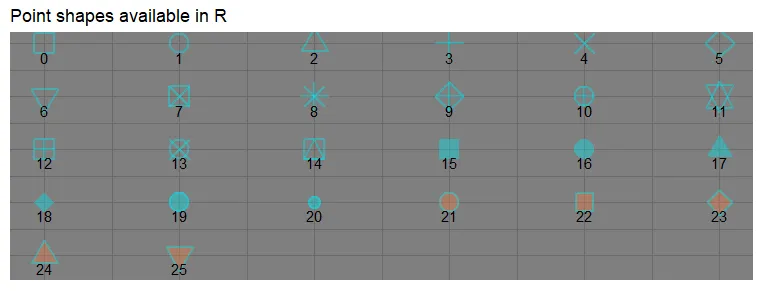In this way, you might see more clearly that in the scenario when you want to get a transparent point without a border, the best option is number 16.

## Change the marker shape in the ggplot2

Here is an example with a scatter plot. By default, the data point contains a tiny border that might not be necessary.

```ggplot(iris, aes(x = Sepal.Length, y = Sepal.Width, color = Species)) +
geom_point(size = 3, alpha = 0.5) +
scale_color_brewer(palette = "Dark2") +
theme_minimal()```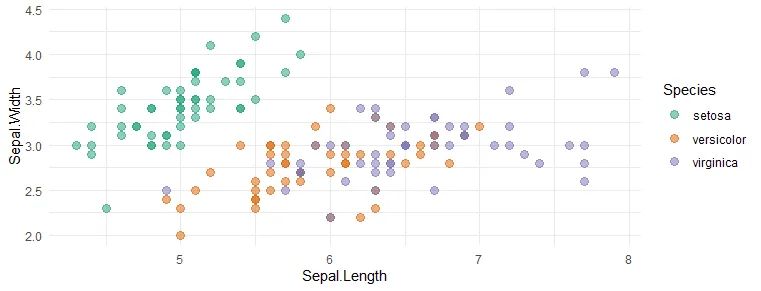The shape of the point without a border in the ggplot2 scatter plot looks like this. You can get that by adjusting the shape argument.

```ggplot(iris, aes(x = Sepal.Length, y = Sepal.Width, color = Species)) +
geom_point(size = 3, alpha = 0.5, shape = 16) +
scale_color_brewer(palette = "Dark2") +
theme_minimal()```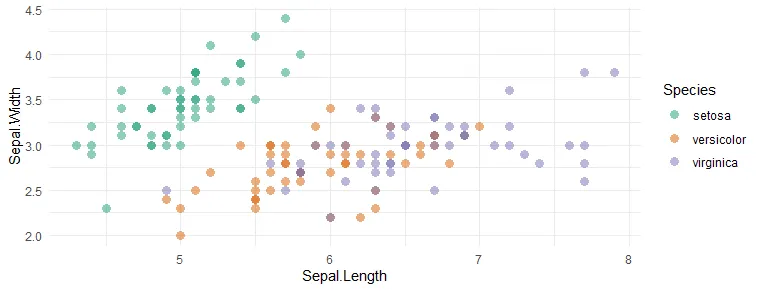The ggplot2 might contain a wide variety of symbols that you can copy and paste inside that argument. For example, here is the R scatter plot with a data point in the shape of a flower.

```ggplot(iris, aes(x = Sepal.Length, y = Sepal.Width, color = Species)) +
geom_point(size = 8, alpha = 0.5, shape = "✿") +
scale_color_brewer(palette = "Dark2") +
theme_minimal()```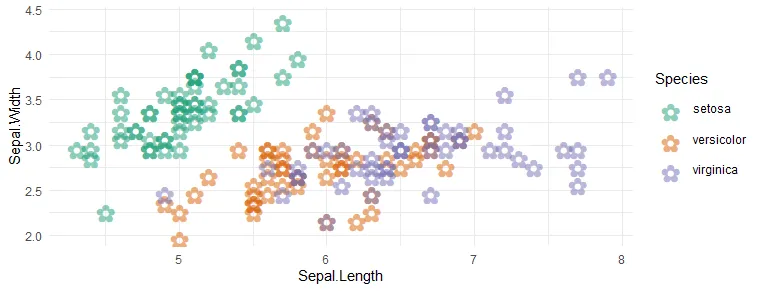## Change the marker shape in the base R

To change how the shape of the data point looks in the plot in the base R, try to use the pch parameter.

```plot(
x = iris\$Sepal.Length, y = iris\$Sepal.Width,
pch = 21,
cex = 1.5,
lwd = 2,
col = "sky blue",
bg = "light gray",
frame.plot = FALSE
)```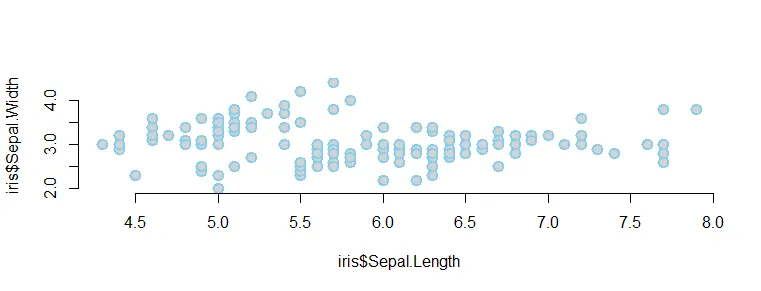## Change the data point shapes in the R plotly

If you want to change the data point shape in the R plotly and you are good at ggplot2, try to use the ggplotly function.

Otherwise, here is how to change marker shape in plotly by using symbol argument.

```require(plotly)

plot_ly(
iris,
x = ~ Sepal.Length,
y = ~ Sepal.Width,
type = "scatter",
mode = "markers",
marker = list(
color = 'LightSkyBlue',
size = 20,
symbol = 22,
line = list(color = 'MediumPurple',
width = 2)
)
)```